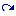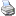# String Operators

 (cached)backlinks... Arithmetic Operators CindyScript CSFundamentals Table of Contents English 日本語

## String Operators

This section lists operators that take strings as arguments. Moreover, operators that generate strings from other values are treated as well.

### Elementary String Operations

#### String concatenation: <string1> + <string2>

Description: The + operator can also be used to append one string to another. The result of such an operation is again a string. If in an addition operation at least one of the arguments is a string, then the other argument will be automatically converted to a string representation of its value.

 Code Result "Cindy"+"Script" "CindyScript" "Four plus three is "+(4+3) "Four plus three is 7" ""+(4+3) "7"

See also: The + operator is also used for the addition of usual numerical values. For this see Arithmetic Operators.

#### Conversion to string: text(<expr>)

Description: The operator text(<expr>) evaluates the expression <expr> and converts the result to a string representation.

#### Length of a string: length(<string>)

Description: This operator returns the number of characters in a string.

Example: length("CindyScript") evaluates to the integer 11.

#### Extracting a substring: substring(<string>,<int1>,<int2>)

Description: This operator returns the substring of <string> that begins after the character indexed by the integer <int1> and ends with the character indexed by <int2>.

Example: substring("abcdefg",3,6) evaluates to the string "def".

#### Searching for occurrence: indexof(<string1>,<string2>)

Description: This operator searches for the first occurrence of <string2> in <string1>. The index of this first occurrence is returned. If <string2> is not a substring of <string1>, then the value 0 is returned.

 Code Result indexof("CindyScript","i") 2 indexof("CindyScript","y") 5 indexof("CindyScript","z") 0

#### Searching for occurrence: indexof(<string1>,<string2>,<int>)

Description: This operator searches for the first occurrence of <string2> in <string1> after the index i. The index of this first such occurrence is returned. If <string2> does not occur in <string1> after index i., then the value 0 is returned.

 Code Result indexof("CindyScript","i",1) 2 indexof("CindyScript","i",3) 9 indexof("CindyScript","i",10) 0

#### Dissecting a string: tokenize(<string>,<expr>)

Description: This operator is very useful for parsing input. It creates a list of substrings of <string>. The second argument <expr> must be either a string or a list of strings. If <expr> is a string, then the operator searches for occurrences of this string in <string>. These occurrences serve as markers for breaking up <string> into a list of pieces.

If <expr> is a list of strings, then a hierarchical list is generated that represents the subdivision of <string> recursively by the tokens in the list.

tokenize usually converts string representations of numbers to number objects. This can lead to information loss. To turn off this behavior, use the autoconvert modifier and set it to false.

 Code Result tokenize( "one:two..three:four", ":") ["one", "two..three", "four"] tokenize( "one:two..three:four", ".") ["one:two", "", "three:four"] tokenize( "one:two..three:four", "..") ["one:two", "three:four"] tokenize( "one:two..three:four", [".",":"]) [["one", "two"], [""], ["three", "four"]] tokenize( "one:two..three:four", ["..",":"]) [["one", "two"], ["three", "four"]] tokenize( "77777777777777777",":")  tokenize( "77777777777777777",":",autoconvert->false) 

#### Replacing in strings: replace(<string1>,<string2>,<string3>)

Description: This operator replaces all (!) occurrences of <string2> in <string1> by <string3>.

This operator is extremely useful for creating text replacement systems of the kind they are used in so called Lindenmeyer Systems.

 Code Result replace( "one:two..three:four", "o", "XXX") "XXXne:twXXX..three:fXXXur" replace("F", "F", "F+F") "F+F" replace("F+F", "F", "F+F") "F+F+F+F"

#### Replacing in strings: replace(<string>,<list>)

Description: This operator is very similar to the previous one. <list> contains a list of replacement pairs, and all such replacements are applied simultaneously to <string>.

 Code Result replace("XYX", [["X","one"], ["Y","two"]]) "onetwoone"

#### Parsing a string: parse(<string>)

Description: This operator parses a string to an expression and evaluates this expression. This operator is particularly useful in processing user input that comes from text fields in a construction.

 Code Result parse("3+7") 10

The code fragment

text="sin(x)+cos(x)";
f(x):=parse(text);


defines the function f(x) to be sin(x)+cos(x).

#### Guessing a good representation of a number: guess(<number>)

Description: This very powerful operator is described in detail in the section Calculus. It takes a numerical expression in floating-point representation and attempts to convert it to a mathematical expression that generates that floating-point number with high precision. This expression is then represented as a string.

 Code Result guess(8.125) "65/8" guess(0.774596669241483) "sqrt(3/5)"

#### Formating a number to a specified precision: format(<number>,<int>)

Description: This operator takes a number as first arguments and an integer specifying the number of digits after the decimal point. A string is generated that corresponds to the number up to the specified precision. Up to 14 digits are possible. If the argument of format is a list of objects the format statement is applied to each of the objects recursively.

 Code Result format(sqrt(2),4) "1.4142" format(pi,14) "3.14159265358979" format([sin(30°),cos(30°)],3) [0.5,0.866]

Warning: The format statement should only produced to create formatted output elements. The formatted values will always be *strings* and hence usually not valid objects for arithmetic operations. The following example illsustrates this:

 Code Result format(sqrt(2),4)+ format(sqrt(2),4) "1.41421.4142"

## String Comparison and Sorting

Like real numbers, strings admit a total ordering. Thus they can be compared using the operators >, <, >=, <=, ==, and !=. Please refer to Boolean Operators for the use of these relations.

The order that is used for strings is lexicographic (dictionary) order. Thus, for example,

"a"<"abd"<"abe"<"b"<"blue"<"blunt"<"xxx"

#### Sorting of lists: sort(<list>,<var>,<expr>)

Description: The various versions of the sort-operator can be used to sort lists that contain string values. The sorting order is usually taken to be the lexicographic order of the words. Alternatively, one can specify a user-defined sorting function such as the lengths of the strings.

 Code Result sort(["one", "two", "three", "four", "five"]) ["five","four","one","three","two"] sort(["one", "two", "three", "four", "five"],length(#)) ["one","two","four","five","three"]

### Accessing and Replacing Characters

#### Index operator: <string>_<int>

Description: The infix operator _, which accesses the fields of a list, can be also used to access a character at a specific position in a string. Characters can be returned and set with this operator.

 Code Result "CindyScript"_5 "y" "CindyScript"_12 undefined

After evaluating the code fragement

a="CindyScript";
a_5="erella";


the variable a contains the string "CinderellaScript".